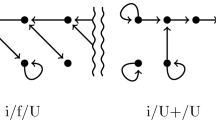# On the Solution Existence of Generalized Quasivariational Inequalities with Discontinuous Multifunctions

## Abstract

We study the following generalized quasivariational inequality problem: given a closed convex set X in a normed space E with the dual E *, a multifunction $$\Phi :X\rightarrow 2^{E^{*}}$$ and a multifunction Γ:X→2X, find a point $$(\hat{x},\hat{z})\in X\times E^{*}$$ such that $$\hat{x}\in \Gamma(\hat{x}),\hat{z}\in \Phi (\hat{x}),\langle \hat{z},\hat{x}-y\rangle \leq 0$$ , $$\forall y\in \Gamma(\hat{x})$$ . We prove some existence theorems in which Φ may be discontinuous, X may be unbounded, and Γ is not assumed to be Hausdorff lower semicontinuous.

This is a preview of subscription content, log in via an institution to check access.

Price excludes VAT (USA)
Tax calculation will be finalised during checkout.

### Similar content being viewed by othersChristoph Tietz

### Subdifferential of the supremum function: moving back and forth between continuous and non-continuous settings

R. Correa, A. Hantoute & M. A. López

Vy Khoi Le

## References

1. Chan, D., Pang, J.S.: The generalized quasivariational inequality problem. Math. Oper. Res. 7, 211–222 (1982)

2. Cubiotti, P.: Finite-dimensional quasivariational inequalities associated with discontinuous functions. J. Optim. Theory Appl. 72, 577–582 (1992)

3. Cubiotti, P.: An existence theorem for generalized quasivariational inequalities. Set-Valued Anal. 1, 81–87 (1993)

4. Cubiotti, P., Yen, N.D.: A result related to Ricceri’s conjecture on generalized quasivariational inequalities. Arch. Math. 69, 507–514 (1997)

5. Cubiotti, P.: Generalized quasivariational inequalities without continuities. J. Optim. Theory Appl. 92, 477–495 (1997)

6. Cubiotti, P.: On the discontinuous infinite-dimensional generalized quasivariational inequality problem. J. Optim. Theory Appl. 115, 97–111 (2002)

7. Cubiotti, P.: Existence theorem for the discontinuous generalized quasivariational inequality problem. J. Optim. Theory Appl. 119, 623–633 (2003)

8. Cubiotti, P., Yao, J.C.: Discontinuous implicit quasivariational inequalities with applications to fuzzy mappings. Math. Methods Oper. Res. 46, 213–328 (1997)

9. Yao, J.C., Guo, J.S.: Variational and generalized variational inequalities with discontinuous mappings. J. Math. Anal. Appl. 182, 371–382 (1994)

10. Yao, J.C.: Generalized quasivariational inequality problems with discontinuous mappings. Math. Oper. Res. 20, 465–478 (1995)

11. Yao, J.C., Guo, J.S.: Extension of strongly nonlinear quasivariational inequalities. Appl. Math. Lett. 5, 35–38 (1992)

12. Yen, N.D.: On an existence theorem for generalized quasivariational inequalities. Set-Valued Anal. 3, 1–10 (1995)

13. Yen, N.D.: On a class of discontinuous vector-valued functions and the associated quasivariational inequalities. Optimization 30, 197–202 (1994)

14. Ricceri, O.N.: On the covering dimension of the fixed-point set of certain multifunctions. Comment. Math. Univ. Carolinae 32, 281–286 (1991)

15. Aubin, J.P., Frankowska, H.: Set-Valued Analysis. Birkhäuser, Basel (1990)

16. Marano, S.A.: Controllability of partial differential inclusions depending on a parameter and distributed-parameter control processes. Le Matematiche 45, 283–300 (1960)

17. Michael, E.: Continuous Selections I. In: Annals of Mathematics, vol. 63, pp. 361–382 (1956)

## Author information

Authors

### Corresponding author

Correspondence to J. C. Yao.

Communicated by X.Q. Yang.

The authors express their sincere gratitude to the referees for helpful suggestions and comments.

This research was partially supported by a grant from the National Science Council of Taiwan, ROC.

B.T. Kien was on leave from National University of Civil Engineering, Hanoi, Vietnam.

## Rights and permissions

Reprints and Permissions

Kien, B.T., Wong, N.C. & Yao, J.C. On the Solution Existence of Generalized Quasivariational Inequalities with Discontinuous Multifunctions. J Optim Theory Appl 135, 515–530 (2007). https://doi.org/10.1007/s10957-007-9239-4